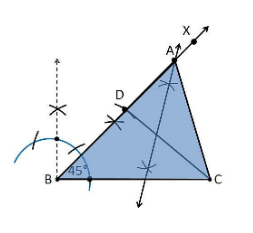Guru

# Construct a triangle ABC in which BC = 8cm, ∠B = 45° and AB–AC = 3.5 cm. Q.2

• 0

How i find the best tricky way for solving the question of class 9th of Constructions chapter of class 9th give me the best and easy method for this question no.2 of exercise 11.2   Construct a triangle ABC in which BC = 8cm, ∠B = 45° and AB–AC = 3.5 cm.

Share

1. Construction Procedure:

The steps to draw the triangle of given measurement is as follows:

1. Draw a line segment of base BC = 8 cm

2. Measure and draw ∠B = 45° and draw the ray BX

3. Take a compass and measure AB-AC = 3.5 cm.

4. With B as centre and draw an arc at the point be D on the ray BX

5. Join DC

6. Now draw the perpendicular bisector of the line CD and the intersection point is taken as A.

7. Now join AC

8. Therefore, ABC is the required triangle.• 0